# Interactions of Optical Solitons

Compatibility:

This lesson demonstrates the particle-like nature of the solitons through some basic features of their interactions.

The fundamental optical soliton propagates (see “Self-phase modulation and group velocity dispersion” from the Tutorials) undistorted due to the exact balance between the nonlinear (SPM) and dispersive (GVD) effects. Thus the soliton propagates as a “particle” (i.e. maintains its shape) rather than as a linear wave packet.

The particle-like nature of solitons manifests itself clearly through the interactions between neighboring solitons. Soliton pulses remain unaffected after interacting with each other. Besides, soliton interaction is phase-sensitive. In-phase solitons “attract” π – but out-of-phase ones repel each other. The intermediate cases (phase difference between zero and π) pertain to an initial attraction followed by repulsion.

When two identical, in-phase solitons, separated in time by T, interact with each other, the time separation between them evolves periodically and the period (the collision length) given by the inverse scattering theory  is: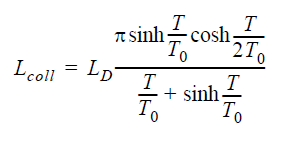In Equation 1, LD is the dispersion length, and T0 is the pulse width. At 40 Gb/s, and
0.5 bit pulse width for sech pulses, T0 = 7.0902ps.

The dispersion length is then: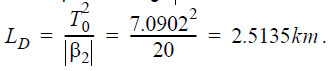With time separation of 75 ps chosen here from Equation 1, we see that the collision
length is Lcoll ≈  782km.

To demonstrate the periodic behavior of soliton interactions we create the following layout (Figure 1).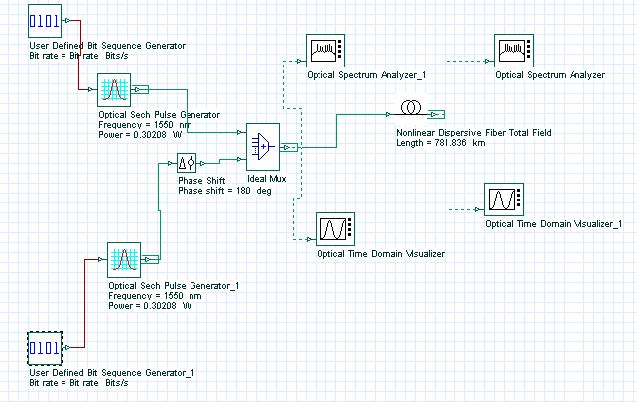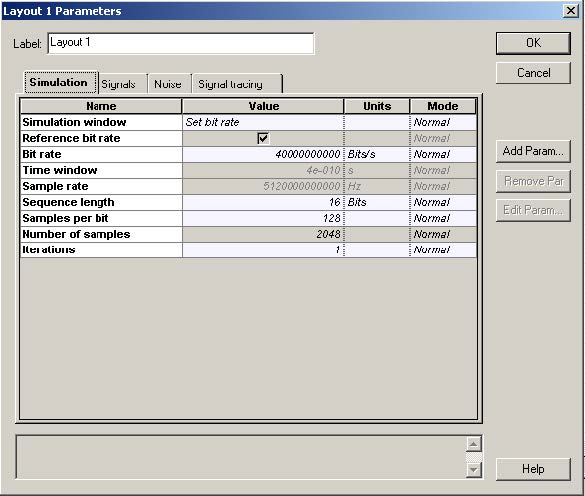Figure 1: The setup to observe soliton interactions

To achieve the necessary accuracy we use the iterative implementation of the split-step Fourier method (Figure 2).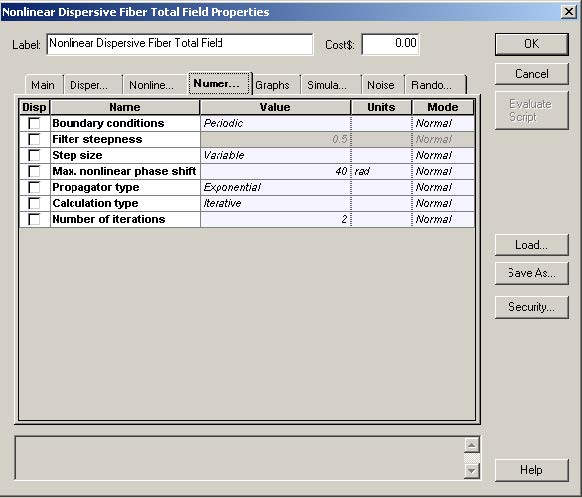Figure 2: Iterative implementation of the split-step Fourier method

At first we consider interaction between two identical in-phase solitons. In this case they form a bound pair.

Figure 3 shows the evolutions of the soliton pair separated initially by 75 ps within one collision length (782 km in this case). The solitons attract each other and become superimposed at a distance equal to half the collision length. Then at a distance equal to the collision length, the initial configuration is restored completely (the pulses are again separated by 75 ps in time).

This picture repeats itself with further propagation with the initial configuration being restored at fiber length equal to multiples of the collision length.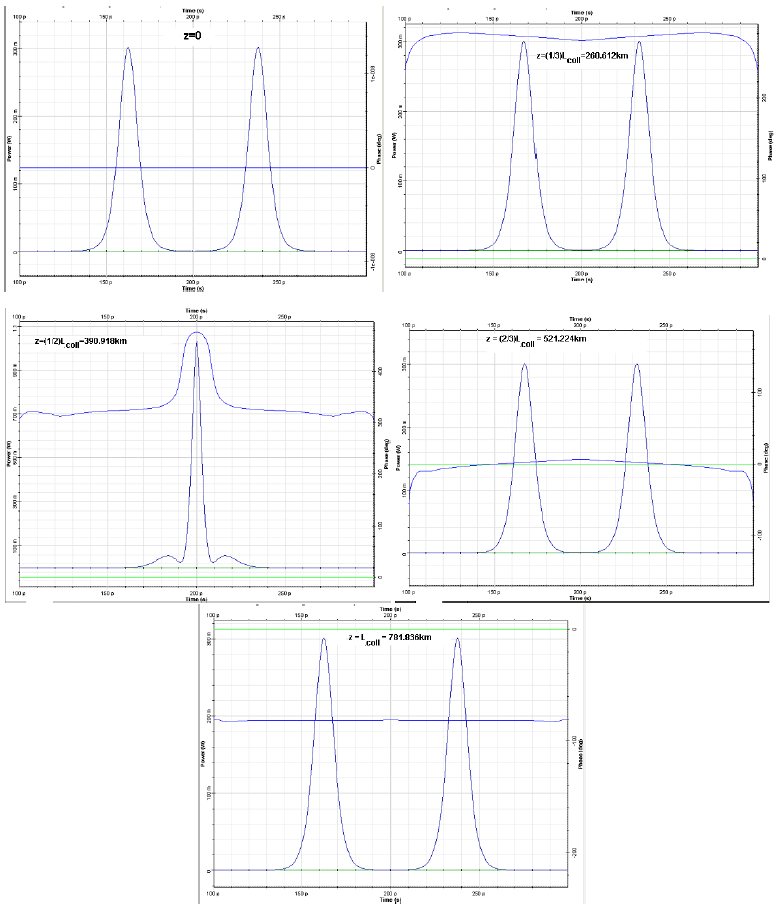Figure 3: Interaction between identical, in-phase solitons within one collision length

The second case we consider is an interaction of an π out-of-phase soliton pair. In this case the time separation TZ after propagation in a fiber with length equal to z can be obtained by treating the interaction as a perturbation, an assumption that is valid in the case of weak overlap between the neighboring solitons . The result is: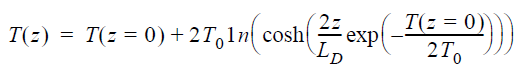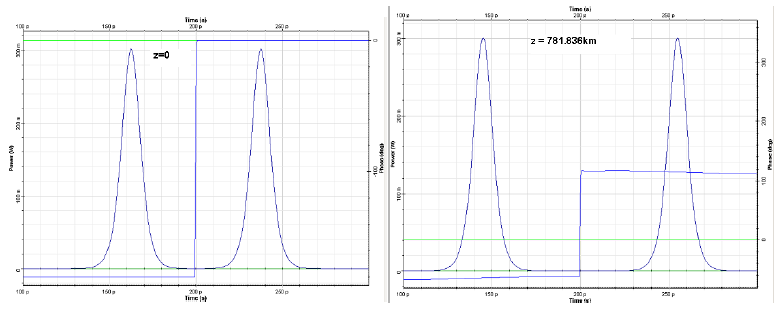Figure 4: Interaction of identical, π-out of phase solitons. Repulsion is evident.

Table 1 compares the results obtained from the perturbation theory, (Equation 1), and those calculated using the layout shown in Figure 1. A good agreement between both is observed.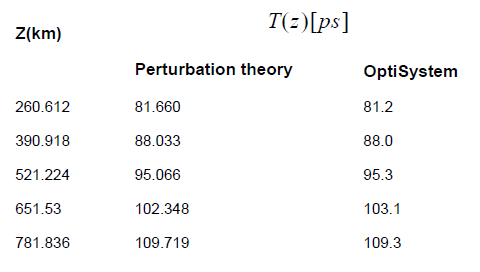Table 1: Perturbation theory results

Reference:
 G. P. Agrawal Nonlinear Fiber Optics, Academic Press (2001).